```SELECTED PRINCIPLES: REDOX REACTIONS (1)
The word redox is a contraction of 'reduction-oxidation'. Historically,
oxidation was used to describe the addition of oxygen to a reactant;
e.g., in this first reaction, magnesium has been oxidized by dioxygen
(which is acting as the oxidizing agent or oxidant):```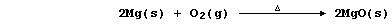```Conversely, reduction described the removal of oxygen from a reactant;
e.g., in this second reaction, copper(II) oxide has been reduced by
dihydrogen (which is acting as the reducing agent or reductant):```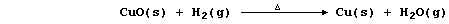```Close inspection of the above reactions reveals two important points.
First, when one reactant is reduced, the other is oxidized (... redox).
And second, when a particle is oxidized it loses electrons, and when a
particle is reduced it gains electrons; e.g.,```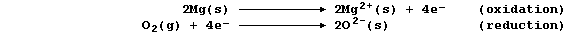```This second point focuses on electron transfer in redox reactions, and
this is the basis for one modern definition of oxidation and reduction:
namely, oxidation is the loss of electrons (Olé!), and reduction is the
gain of electrons.
Most recently, another definition of oxidation and reduction has found
widespread favour: namely, one based on changes in oxidation numbers
(often called oxidation states). Because a comprehensive presentation
of oxidation numbers is beyond the scope of this text, a brutal summary
will have to suffice: oxidation is an increase in oxidation number, and
reduction is a decrease in oxidation number ... as illustrated below.```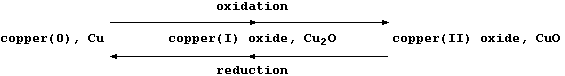```        The oxidation number or state, (in Roman numerals), is
placed immediately after the name of the element concerned```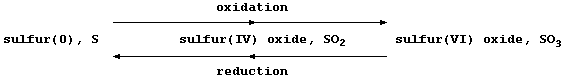`     I = 1   II = 2   III = 3   IV = 4   V = 5   VI = 6   VII = 7`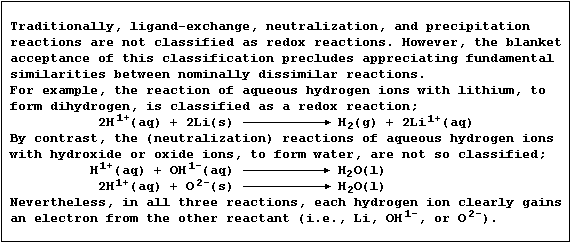```1.  Oxidizing agents are substances which cause oxidation; examples of
these reagents used in laboratories include potassium dichromate(VI),
concentrated tetraoxosulfuric(VI) acid, and potassium manganate(VII).
(a) Potassium dichromate(VI), dissolved in aqueous sulfuric acid, is a
convenient reagent for the oxidation of alcohols to their corresponding
carboxylic acids; e.g., propan-1-ol is oxidized to propanoic acid:```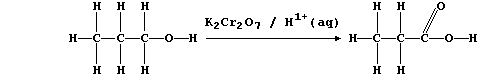```In this reaction, the orange dichromate(VI) ions are reduced to green
chromium(III) ions; a simplified redox half equation is as follows:```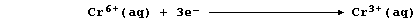```Recalling that the number of electrons equals the number of protons in
a neutral atom of an element, state the number of electrons there are
in each of these particles:
24Cr __________   24Cr3+ __________   24Cr6+ __________   18Ar ___________

(b) Concentrated tetraoxosulfuric(VI) acid, better known as sulfuric
acid, oxidizes hydrogen bromide as follows:```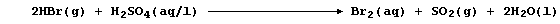```State the change in oxidation number of sulfur. _______________________

Suggest two observations which should be made during this reaction. ___
_______________________________________________________________________

(c) Potassium manganate(VII), dissolved in aqueous potassium hydroxide,
is a reagent sometimes used to test for the presence of a C=C double
bond; e.g., cis-but-2-ene is oxidized to butane-2,3-diol:```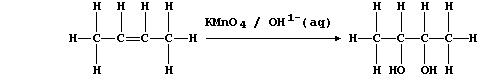```In this reaction, the purple manganate(VII) ions are reduced to brown
manganese(IV) ions; a simplified redox half equation is as follows:```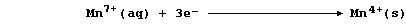```State the number of electrons there are in each of these particles:
25Mn __________   25Mn4+ __________   25Mn7+ __________   18Ar ___________

2.  Reducing agents are substances which cause reduction; examples of
these reagents used in laboratories include magnesium(s), methane(g),
and carbon-graphite(s). Construct a symbol equation for the reduction
of lead(IV) oxide to lead(0) with each of these named reducing agents.
_______________________________________________________________________
_______________________________________________________________________
_______________________________________________________________________

3.  Lead(II) ions are very toxic to most living organisms because they
inhibit the 'active sites' of a wide variety of enzymes; in particular,
those which are catalysts in the biosynthesis of compounds involved,
directly or indirectly, in the redox processes of either photosynthesis
or cellular respiration or both (e.g., chlorophylls, cytochromes, and
haemoglobin). Name the condition caused by a shortage of haemoglobin,
the dioxygen-carrying pigment in mammalian red blood cells. ___________

Dr. R. Peters                   Next                     Contents' List```